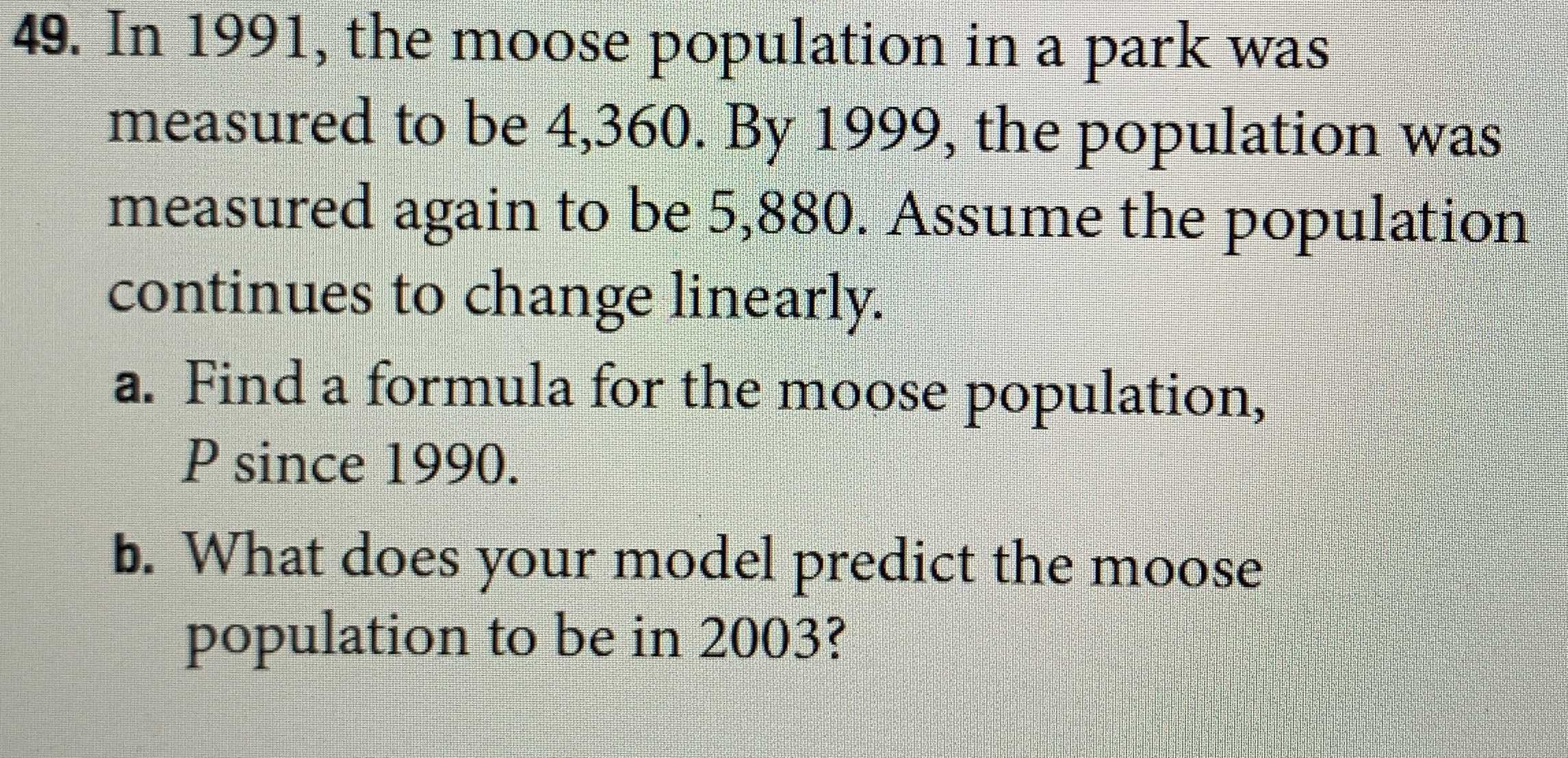### ¿Todavía tienes preguntas de matemáticas?

Pregunte a nuestros tutores expertos
Algebra
Pregunta49. In $$1991$$ , the moose population in a park was measured to be $$4,360$$ . By $$1999$$ , the population was measured again to be $$5,880$$ . Assume the population continues to change linearly. a. Find a formula for the moose population, $$P$$ since $$1990$$ . b. What does your model predict the moose population to be in $$2003$$ ?